## 12.3.2 Stentz's Algorithm (D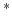)

Imagine exploring an unknown planet using a robotic vehicle. The robot moves along the rugged terrain while using a range scanner to make precise measurements of the ground in its vicinity. As the robot moves, it may discover that some parts were easier to traverse than it originally thought. In other cases, it might realize that some direction it was intending to go is impassable due to a large bolder or a ravine. If the goal is to arrive at some specified coordinates, this problem can be viewed as a navigation problem in an unknown environment. The resulting solution is a lazy approach, as discussed in Section 12.2.1.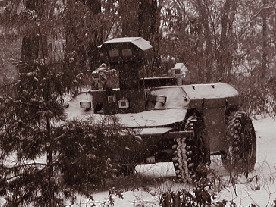This section presents Stentz's algorithm , which has been used in many outdoor vehicle navigation applications, such as the vehicle shown in Figure 12.17. The algorithm can be considered as a dynamic version of the backward variant of Dijkstra's algorithm. Thus, it maintains cost-to-go values, and the search grows outward from the goal, as opposed to cost-to-come values from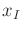in the version of Dijkstra's algorithm in Section 2.3.3. The method applies to any optimal planning problem. In terms of the state transition graph, it is assumed that the costs of edge transitions are unknown (equivalently, each cost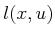is unknown). In the navigation problem, a positive cost indicates the difficulty of traveling from stateto state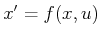.

To work with a concrete problem, imagine that a planet surface is partitioned into a high-resolution grid. The state space is simply a bounded set of grid tiles; hence,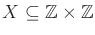. Each grid tile is assigned a positive, real value,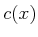, that indicates the difficulty of its traversal. The actions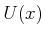at each grid point can be chosen using standard grid neighbors (e.g., four-neighbors or eight-neighbors). This now defines a state transition graph over. From any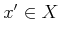and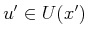such that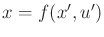, the cost term is assigned using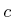as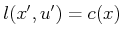. This model is a generalization of the grid in Section 12.3.1, in which the tiles were either empty or occupied; here any positive real value is allowed. In the coming explanation, the costs may be more general than what is permitted by starting from, and the state transition graph does not need to be derived from a grid. Some initial values are assigned arbitrarily for all. For example, in the planetary exploration application, the cost of traversing a level, unobstructed surface may be uniformly assumed.

The task is to navigate to some goal state,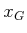. The method works by initially constructing a feedback plan,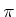, on a subset ofthat includes both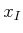and. The plan,, is computed by iteratively applying the procedure in Figure 12.18 until the optimal cost-to-go is known at. A priority queue,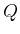, is maintained as in Dijkstra's algorithm; however, Stentz's algorithm allows the costs of elements into be modified due to information sensed during execution. Let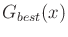denote the lowest cost-to-go associated withduring the time it spends in. Assume thatis sorted according to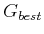. Let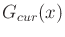denote its current cost-to-go value, which may actually be more thanif some cost updates caused it to increase. Suppose that some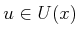can be applied to reach a state. Let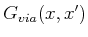denote the cost-to-go fromby traveling via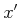,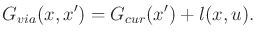(12.24)

If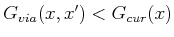, then it indicates thatcould be reduced. If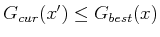, then it is furthermore known that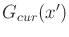is optimal. If both of these conditions are met, thenis updated to.

After the iterations of Figure 12.18 finish, the robot executes, which generates a sequence of visited states. Let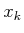denote the current state during execution. If it is discovered that if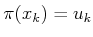would be applied, the received cost would not match the cost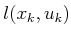in the current model, then the costs need to be updated. More generally, the robot may have to be able to update costs within a region aroundthat corresponds to the sensor field of view. For the description below, assume that an update,, is obtained foronly (the more general case is handled similarly). First,is updated to the newly measured value. Ifhappened to be dead (visited, but no longer in), then it is inserted again into, with cost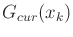. The steps in Figure 12.18 are performed until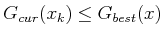for all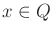. Following this, the plan execution continues until either the goal is reached or another cost mismatch is discovered. At any time during execution, the robot motions are optimal given the current information about the costs .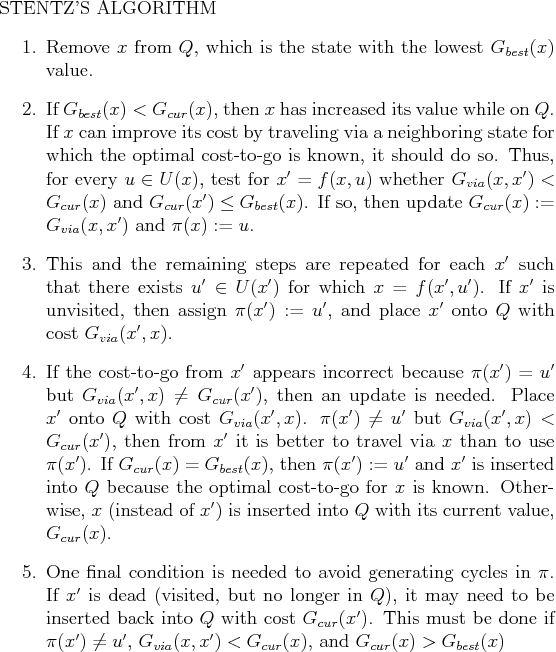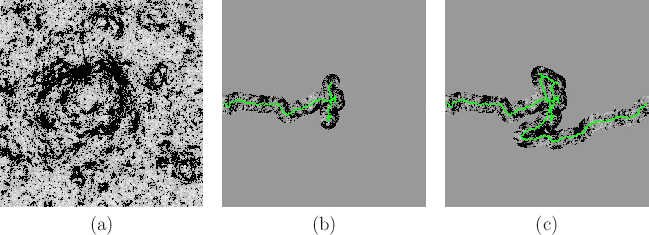Figure 12.19 illustrates the execution of the algorithm. Figure 12.19a shows a synthetic terrain that was generated by a stochastic fractal. Darker gray values indicate higher cost. In the center, very costly terrain acts as a barrier, for which an escape route exists in the downward direction. The initial state is the middle of the left edge of the environment, and the goal state is the right edge. The robot initially plans a straight-line path and then incrementally updates the path in each step as it moves. In Figure 12.19b, the robot has encountered the costly center and begins to search for a way around. Finally, the goal is reached, as shown in Figure 12.19c. The executed path is actually the result of executing a series of optimal paths, each of which is based on the known information at the time a single action is applied.

Subsections
Steven M LaValle 2020-08-14